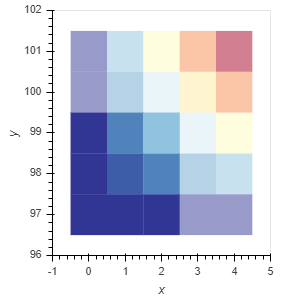# Holoviews Heatmap specify color for each point

I’m trying to generate a Heatmap in Holoviews with custom color values for each point, in order to specify different alpha color values.

Currently I’m generating two Holoviews plots with different alpha values and overlay them like so:

``````data = pd.DataFrame([(i, 97+j,  i*j) for i in range(5) for j in range(5)],
columns=['x', 'y', 'val'])
data_filtered = data[(data.x < 3) & (data.y < 100)]

hm_opts = dict(kdims=['x', 'y'], vdims=['val'])
hm = hv.HeatMap(data, **hm_opts).opts(alpha=0.5)
hm_filtered = hv.HeatMap(data_filtered, **hm_opts).opts()
hm * hm_filtered
``````This however, gives me a Holoviews Overlay object, where I’d like to have a single HeatMap object instead.

My idea was to map each x/y coordinate to a specific color value in hex form, which has the needed alpha already calculated into it. My DataFrame would therefore look something like this (example):

``````    x    y  val    color
0   0   97    0  #00FF00
1   0   98    0  #00FF00
2   0   99    0  #00FF00
...
22  4   99    8  #FFD29F
23  4  100   12  #FFB89F
24  4  101   16  #D3AFF4
``````

Is there a way to tell Holoviews to use these colors?
When I pass the list of colors to the “cmap” parameter it interpretes it as color intervals, passing the name of the column raises an error as it cannot find the specified cmap.

When I pass the list to the “color” parameter, the plot is not displayed at all anymore in the Jupyter Notebook.

I also posted this question at StackOverflow.

Thanks for this post, @iRave! I came to ask a nearly identical question and the answer on StackOverflow enabled me to figure out how to customize my plot (I was trying to specify transparency and color for a boolean array).

1 Like

On stackover flow. I answer in https://stackoverflow.com/a/64469912/4989371

Hope this help

Thanks, this certainly helped. I updated my code on StackOverflow with the help of your answer.

So I recently discovered an odd behavior using the color ranges based solution proposed on stackoverflow. Now I try to align the colormaps of multiple heatmap plots, which should be possible by supplying the same color intervals. Therefore, the maximum value of the color intervals does not necessarely match the maximum value in the heatmap, which sometimes leads to an odd behavior where a half open interval suddenly becomes a closed interval.

Below, you find the example given in the stackoverflow post where I adjust the “max_value” in order to get different interval bounds.
My goal is to have a color mapping where [0, 1) = green, [1, max_count / 2) = yellow, [max_count / 2, max_count) = red and max_count = violet.
However, with the first interval I get [0,1] (inclusive), where ‘1’ is part of the green color, whenever max_value reaches a specific threshold (in this example 23). Is this expected and if so, is there a way around that?

data = pd.DataFrame([(i, 97+j, i*j) for i in range(5) for j in range(5)], columns=[‘x’, ‘y’, ‘val’])
max_count = 23
# [0, 1, 11, 22, 23, 24]
# max_count = data.val.max()
# [0, 1, 8, 15, 16, 17]
red = ‘#FF0000
yellow = ‘#FFFF00
green = ‘#00FF00
blue_violet = ‘#8A2BE2
levels = [0, 1, int(max_count / 2), max_count - 1, max_count, max_count + 1]
colors = [green, yellow, red, blue_violet, blue_violet]
hv.HeatMap(data2).opts(width=900, height=400, cmap=colors, color_levels=levels, line_width=1).opts(colorbar=True)

if everything else fails consider something like this

``````def paint(x, max_count):
if x < 1:
return 10 #green
elif x <  int(max_count / 2):
return 20 #yellow
elif x <  (max_count - 1):
return 30 #red
elif x <  (max_count):
return 40 # blue_violet
else:
return 40 # blue_violet
data['color_tr'] = data.apply(lambda x: paint(x['val'], 23), axis=1)
levels = [10, 19, 29, 39, 49]
colors = [green, yellow, red, blue_violet]

hv.HeatMap(data,kdims=['x', 'y'], vdims=['color_tr','val']).opts(
width=900, height=400, line_width=1, cmap=colors, color_levels=levels, tools=['hover'])
``````

probably you must remove the colorbar…

1 Like